• 任务：为宿舍管理人员编写一个宿舍管理查询软件, 程序设计要求：采用交互工作方式 建立数据文件 ，数据文件按关键字（姓名、学号、房号）进行排序(冒泡、选择、插入排序等任选一种)，文件中数据记录不少于10条 查询...
• 为宿舍管理人员编写一个宿舍管理查询软件，附有源码和文档
• 数据结构课程设计 宿舍管理查询软件 家31大嶂秦皇岛分校 NorthEastern University At QinHuangDao 数据结构课程设计 宿舍管理查询软件 班级学号 班 学生姓名 提交日期 7月24日 成 绩 计算机与通信工程学院 一需求...
• 数据结构课程设计 宿舍管理查询软件 班 班级学号 学生姓2012提交日 绩 计算机与通信工程学院 一需求分析 1程序设计任务 为宿舍管理人员编写一个宿舍管理查询软件 程序设计要求 A. 采用交互工作方式 B. 建立数据文件 ...
• 某高校学生的住宿安排比较灵活，一个学院有可能住在不同的宿舍楼，为方便学生管理者快速查找学生的住宿信息，设计一个小型的宿舍管理查询软件。其中学生信息包括：学号、姓名、学院、楼号、房间号。 一堆废话 很久...
问题描述
某高校学生的住宿安排比较灵活，一个学院有可能住在不同的宿舍楼，为方便学生管理者快速查找学生的住宿信息，设计一个小型的宿舍管理查询软件。其中学生信息包括：学号、姓名、学院、楼号、房间号。
一堆废话
很久没有更新博客了，小白刚刚历经考试周的打击，百废待兴，赶紧跑来CSDN和小伙伴们取暖安慰受伤的心灵。近期会陆续写四篇数据结构课设的博客，然后再写两篇编写小游戏的博客，一个是基于Python的飞机大战，另一个是基于C++并在funcode上完成的海底世界游戏。
数据描述
（1）首先定义一个结构体，用来存放某个学生的信息，包括学号、姓名、学院、楼号、房间号。
//定义学生信息结构体
typedef struct{
int number;
char name;
char college;
int building;
int room;
}Student;

（4）再定义一个顺序表，用具有20个元素顺序表来存放20个学生的信息。每个元素分别表示一个药品信息的结构体。
//定义存储顺序表
typedef struct {
Student s;
int length;
}SqList;

程序代码
#include<stdio.h>
#include<stdlib.h>
#include<string.h>
//定义学生信息结构体
typedef struct{
int number;
char name;
char college;
int building;
int room;
}Student;
//定义存储顺序表
typedef struct {
Student s;
int length;
}SqList;
void CreatStudent(SqList &L,int n)//输入学生信息
{
L.length=0;
for(int i=1;i<=n;i++)
{
printf("输入第%d个学生信息\n",i);
printf ("学号:");
scanf("%d",&L.s[i].number);
printf ("姓名:");
scanf("%s",&L.s[i].name);
printf ("学院:");
scanf("%s",&L.s[i].college);
printf ("楼号:");
scanf("%d",&L.s[i].building);
printf ("房间号:");
scanf("%d",&L.s[i].room);
L.length++;
}
FILE *fp;
fp=fopen("room.txt","w+");
for(int i=1;i<=L.length;i++)
{
fprintf(fp,"%d %s %s %d %d\n",L.s[i].number,L.s[i].name,L.s[i].college,L.s[i].building,L.s[i].room);
}
printf("添加成功!\n");
fclose(fp);
}
void NumberSort(SqList &L)//按学号进行排序
{
int i=1;
L.length=0;
FILE *fp1;
fp1=fopen("room.txt","r+");
while(!feof(fp1))
{
fscanf(fp1,"%d %s %s %d %d",&L.s[i].number,L.s[i].name,L.s[i].college,&L.s[i].building,&L.s[i].room);
L.length++;
i++;
}
L.length--;
fclose(fp1);
for(i=2;i<=L.length;i++)
{
if(L.s[i].number<L.s[i-1].number)
{
L.s=L.s[i];
L.s[i]=L.s[i-1];
int j;
for(j=i-2;L.s.number<L.s[j].number;j--)
{
L.s[j+1]=L.s[j];
}
L.s[j+1]=L.s;
}
}
printf("按学号排序为：\n");
for(int i=1;i<=L.length;i++)
{
printf("%d %s %s %d %d\n",L.s[i].number,L.s[i].name,L.s[i].college,L.s[i].building,L.s[i].room);
}
printf("排序成功!\n");
}
void NameSort(SqList &L)//按姓名排序
{
int i=1,j;
L.length=0;
FILE *fp1;
fp1=fopen("room.txt","r+");
while(!feof(fp1))
{
fscanf(fp1,"%d %s %s %d %d",&L.s[i].number,L.s[i].name,L.s[i].college,&L.s[i].building,&L.s[i].room);
L.length++;
i++;
}
L.length--;
fclose(fp1);
for(i=1;i<L.length;i++)
{
for(j=1;j<=L.length-i;j++)
{
if(strcmp(L.s[j].name,L.s[j+1].name)>=0)
{
L.s=L.s[j];
L.s[j]=L.s[j+1];
L.s[j+1]=L.s;
}
}
}
printf("按姓名排序为：\n");
for(int i=1;i<=L.length;i++)
{
printf("学号:%d  姓名:%s  学院:%s  楼号:%d  房间号:%d\n",L.s[i].number,L.s[i].name,L.s[i].college,L.s[i].building,L.s[i].room);
}
printf("排序成功!\n");
} void BuildingSort(SqList &L)//按楼号进行排序
{
int i=1;
L.length=0;
FILE *fp1;
fp1=fopen("room.txt","r+");
while(!feof(fp1))
{
fscanf(fp1,"%d %s %s %d %d",&L.s[i].number,L.s[i].name,L.s[i].college,&L.s[i].building,&L.s[i].room);
L.length++;
i++;
}
L.length--;
fclose(fp1);
for(i=2;i<=L.length;i++)
{
if(L.s[i].building<L.s[i-1].building)
{
L.s=L.s[i];
L.s[i]=L.s[i-1];
int j;
for(j=i-2;L.s.building<L.s[j].building;j--)
{
L.s[j+1]=L.s[j];
}
L.s[j+1]=L.s;
}
}
printf("按楼号排序为：\n");
for(int i=1;i<=L.length;i++)
{
printf("学号:%d  姓名:%s  学院:%s  楼号:%d  房间号:%d\n",L.s[i].number,L.s[i].name,L.s[i].college,L.s[i].building,L.s[i].room);
}
printf("排序成功!\n");
}
void NumberSearch(SqList &L) //按学号查询
{
int i=1,k,flag=0;
int num;
L.length=0;
FILE *fp1;
fp1=fopen("room.txt","r+");
while(!feof(fp1))
{
fscanf(fp1,"%d %s %s %d %d",&L.s[i].number,L.s[i].name,L.s[i].college,&L.s[i].building,&L.s[i].room);
L.length++;
i++;
}
L.length--;
fclose(fp1);
printf("输入要查询学生的学号：\n");
scanf("%d",&num);
for(i=1;i<=L.length;i++)
{
if(L.s[i].number==num)
{
k=i;
flag=1;
}
}
if(flag==0)
printf("没有找到该学生，查询失败！\n");
else
printf("学号:%d  姓名:%s  学院:%s  楼号:%d  房间号:%d\n",L.s[k].number,L.s[k].name,L.s[k].college,L.s[k].building,L.s[k].room);
}
void NameSearch(SqList &L)
{
int i=1,k,flag=0;
char na;
L.length=0;
FILE *fp1;
fp1=fopen("room.txt","r+");
while(!feof(fp1))
{
fscanf(fp1,"%d %s %s %d %d",&L.s[i].number,L.s[i].name,L.s[i].college,&L.s[i].building,&L.s[i].room);
L.length++;
i++;
}
L.length--;
fclose(fp1);
printf("输入要查询学生的姓名：\n");
scanf("%s",na);
for(i=1;i<=L.length;i++)
{
if(strcmp(L.s[i].name,na)==0)
{
k=i;
flag=1;
}
}
if(flag==0)
printf("没有找到该学生，查询失败！\n");
else
printf("学号:%d  姓名:%s  学院:%s  楼号:%d  房间号:%d\n",L.s[k].number,L.s[k].name,L.s[k].college,L.s[k].building,L.s[k].room);
}
void BuildingSearch(SqList &L)
{
int i=1,flag=0;
int bu;
L.length=0;
FILE *fp1;
fp1=fopen("room.txt","r+");
while(!feof(fp1))
{
fscanf(fp1,"%d %s %s %d %d",&L.s[i].number,L.s[i].name,L.s[i].college,&L.s[i].building,&L.s[i].room);
L.length++;
i++;
}
L.length--;
fclose(fp1);
printf("输入要查询学生的楼号：\n");
scanf("%d",&bu);
for(i=1;i<=L.length;i++)
{
if(L.s[i].building==bu)
{
printf("学号:%d  姓名:%s  学院:%s  楼号:%d  房间号:%d\n",L.s[i].number,L.s[i].name,L.s[i].college,L.s[i].building,L.s[i].room);
flag=1;
}
}
if(flag==0)
printf("没有找到该楼号，查询失败！\n");
}
void ShowRoom(SqList &L)
{
int i=1;
L.length=0;
FILE *fp1;
fp1=fopen("room.txt","r+");
while(!feof(fp1))
{
fscanf(fp1,"%d %s %s %d %d",&L.s[i].number,L.s[i].name,L.s[i].college,&L.s[i].building,&L.s[i].room);
L.length++;
i++;
}
L.length--;
fclose(fp1);
for(i=1;i<=L.length+1;i++)
{
printf("学号:%d  姓名:%s  学院:%s  楼号:%d  房间号:%d\n",L.s[i].number,L.s[i].name,L.s[i].college,L.s[i].building,L.s[i].room);
}
}
int main()
{
SqList L;
int n,option;

printf("********欢迎进入宿舍管理查询系统**************\n");
ShowRoom(L);
while(1)
{
printf("****************************************");
printf("\n1.创建\t2.按学号排序\n");
printf("\n3.按姓名排序\t4.按楼号排序\n");
printf("\n5.按学号查询\t6.按姓名查询\n");
printf("\n7.按楼号查询\t8.退出\n");
printf("****************************************");
printf("\n请选择需要的服务:");
scanf("%d",&option);
switch(option)
{
case 1:
printf("请输入学生个数:");
scanf("%d",&n);
CreatStudent(L,n);
break;
case 2:
NumberSort(L);
break;
case 3:
NameSort(L);
break;
case 4:
BuildingSort(L);
break;
case 5:
NumberSearch(L);
break;
case 6:
NameSearch(L);
break;
case 7:
BuildingSearch(L);
break;
case 8:
exit(0);
break;
}
}
return 0;
}

测试数据
（1）开始界面（显示文件信息和系统服务选项）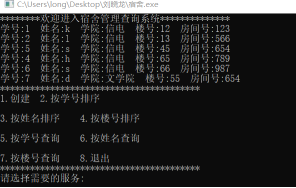（2）按学生学号进行排序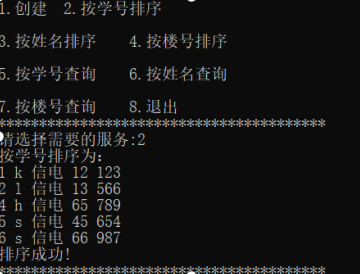（3）按销学生姓名进行排序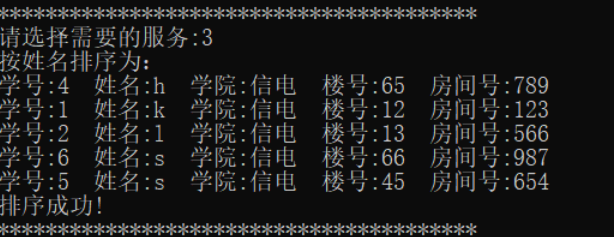（4）按楼号进行排序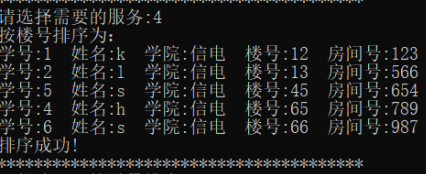（5）按学号查询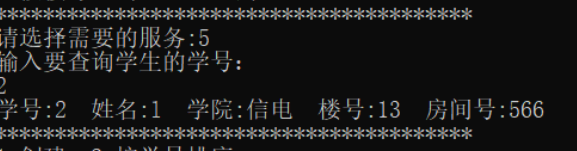（6）按姓名查询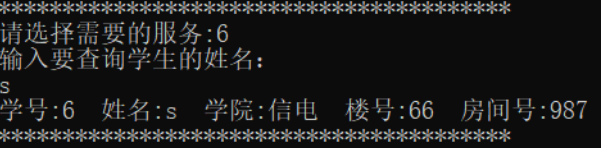（7）按楼号查询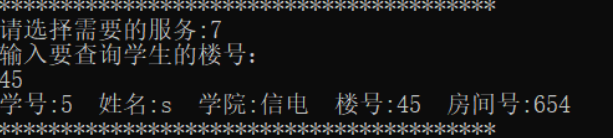展开全文• 景德镇陶瓷大学 ... 编写宿舍管理查询软件 姓 名 杨茹 班 级 17信管一班 学 号 117060300109 完成日期 2018...
景德镇陶瓷大学

实践周课程设计报告

实验名称

编写宿舍管理查询软件

姓    名

杨茹

班    级

17信管一班

学    号

117060300109

完成日期

2018年12月27日

实验目的
为宿舍管理人员编写一个宿舍管理查询软件

设计要求
采用交互工作方式
建立数据文件，数据文件按关键字（姓名、学号、房号）进行排序(冒泡、选择、插入排序等任选一种)
查询菜单(用二分查找实现以下操作)
按姓名查询
按学号查询
按宿舍号查询
打印任一查询结果（可以连续操作）
需求分析
性能分析

宿舍内入住若干学生，每个宿舍都有唯一独立编码，入住学生有唯一的姓名、学号。编写宿舍管理系统，添加学生的姓名、学号、宿舍号为关键字，创建相应函数实现各种排序、查找和插入删除等操作。使用这些关键字及函数方便进行宿舍管理。

功能分析

实现交互工作方式，各项操作结束后均应返回主菜单；
建立数据文件：系统本无任何信息数据，需开发一个“信息输入”功能，即首先创建一个学员线性表，同时我们可以将数据暂时保存在内存中，学生信息包括：姓名，学号，宿舍号；
显示：信息录入后都保存在内存中，用户看不到，需要设计一个信息显示功能，信息的显示应该便于查阅，所以需具备按各种关键字显示的功能；
排序：本系统按关键字（姓名、学号、房号）进行冒泡排序；
查询：采用二分查找方式，分别实现按关键字（姓名、学号、房号）查询功能；
删除:于有些同学因为不同原因而离校，所以设计了删除功能;
插入：由于有新同学入校，所以设计了插入功能；、
打印：将查询到的某一学生信息或全部学生信息打印；
退出：退出交互工作系统。

功能

输入

查询

排序

显示

插入

删除

退出

（图1  功能简图）

用户需求

创建欢迎界面；
创建线性表，提示用户输入信息，并现实显示信息，输出各种关键字信息，便于用户查阅；
创建主界面，建立主选择菜单和子选择菜单，便于用户操作；
创建返回主菜单的函数，人性化设计；
提供推出选项，操作完毕需要退出时，提供选项，便于使用者退出交互工作系统；
优化程序，提供友好界面，操作键如下：
0：退出程序       1：按姓名排序     2：按学号排序

3：按宿舍号排序   4：按姓名查找     5：按学号查找

6：按宿舍号查找   7：按学号插入     8：按学号删除

框架设计

宿舍管理系统功能模块图及流程图

姓名

学号

宿舍号

学

生

信

息

表

按照姓名排序，冒泡排序

按照学号排序，冒泡排序

按照宿舍排序，冒泡排序

排序模块

按照姓名从小到大查找，二分法查找

按照学号从小到大查找，二分法查找

按照宿舍从小到大查找，二分法查找

查找模块

按照学号从小到大插入

按照学号从小到大删除

插入和删除

（图2 功能模块图）

y

1<=f<=8

f<0或f>8

f=0

显示学生信息

是否继续

按姓名排序

按学号排序

按宿舍号排序

按姓名查找

按学号查找

按宿舍号查找

按学号插入

按学号删除

输入

姓名

输入

学号

输入

宿舍号

输入

信息

输入

学号

退出

开始

（主界面）

输入学生信息

是否继续输入

输入整数t

判断t

y

（图 3 系统流程图）

概要设计
存储结构
单链表存储，结构体定义为

typedef struct{           //定义结构体成员

char name;

int num;                 //学号宿舍号为整型

int room;

}stu;

stu stud;

typedef struct{

int Length;              //当前长度

stu *Elem;               //存储空间基址

int ListSize;            //当前分配的存储容量

}LinkList;

数据设计
int f                  全局变量，指选择的操作键数

char name          姓名

int num                学号

int room               宿舍号

int Length            为线性表的当前长度

stu *Elem;          指示线性表的基地址

int Listsize          当前分配的存储容量

char c                 进入主界面的任意键

ch                    判断字符y或n

int i,j               冒泡排序中，i为外层循环次数，j为内层循环次数

int i                打印学生信息时，i作为循环变量

int m;,int n;,int a   m,n,a在二分查找中分别代表房号，学号，姓名

int i,j,k;           i,j,k在按学号插入中作为循环变量

int i,j,k=-1          i,j,k在按学号删除中作为循环变量

函数设计
int menu();                //列出主菜单

void Init(LinkList &L)     //初始化线性表

void Create(LinkList &L)   //创建学生信息表

void Shuru(LinkList L)     //学生信息录入

void Disp()                //返回主界面，调用menu（）函数实现

void Sorte(LinkList &L)    //按照姓名由小到大排序，冒泡排序

void Sortn(LinkList &L)    //按照学号由小到大排序，冒泡排序

void Sortr(LinkList &L)    //按照宿舍号由小到大排序，冒泡排序

void chazhaoe(LinkList &L) //按姓名从小到大查找，二分查找

void chazhaon(LinkList &L) //按学号从小到大查找，二分查找

void chazhaor(LinkList &L) //按宿舍号从小到大查找，二分查找

void Insert(LinkList &L)  //按学号从小到大插入该学生

void Delete(LinkList &L)  //按学号从小到大删除该学生

int panduan2(char ch)   //如果学生不存在,判断是否继续查找，通过if语句，文件操作函数实现

void panduan3()        //如果已无学生记录则返回主界面，通过调用其他函数和文件操作函数实现

void print1 LinkList L）  //打印学生信息，通过调用文件操作函数实现

void print2(LinkList L,int mid)//输出学生信息，通过调用文件查找函数实现

void main()                //主函数，系统操作入口

详细设计
实验源代码如下：

// 17信管宿舍管理系统.cpp : 定义控制台应用程序的入口点。

#include "stdafx.h"

#include<stdio.h>

#include<stdlib.h>

#include<string.h>

#define N 40

#define increase 10

int f,t=0;

typedef struct{

char name;

int  num;

int  room;

}stu;

stu stud;

typedef struct{

int Length;

stu *Elem;

int ListSize;

}LinkList;

void Init(LinkList &L){                                //初始化

L.Length=0;

L.Elem=(stu *)malloc(N * sizeof(stu ));

L.ListSize=N;

}

void menu(){                                      //菜单界面

printf("\n");

printf("----------------------------17信管1班宿舍管理查询系统---------------------------\n");

printf("\n");printf("\n");

printf("                             1、 按姓名排序\n");        printf("\n");

printf("                             2、 按学号排序\n");        printf("\n");

printf("                             3、 按宿舍号排序\n");   printf("\n");

printf("                             4、 按姓名查找\n");        printf("\n");

printf("                             5、 按学号查找\n");        printf("\n");

printf("                             6、 按宿舍号查找\n");   printf("\n");

printf("                             7、 按学号插入\n");        printf("\n");

printf("                             8、 按学号删除\n");

printf("\n");printf("\n");printf("\n");

printf("提示：当输入的数字键为0时，退出操作\n");

if(t==1)

{

printf("请输入数字键（1——8为操作键）：");

scanf_s("%d",&f);

if(f<0||f>9)

{

system("cls");

printf("\n");

printf("输入的数字不对，请在原处重新输入\n");printf("\n");

menu();

}

}

}

void Disp(){                                      //返回主界面

char c;

fflush(stdin);printf("\n");

printf("请按任意键进入主界面：");

scanf_s("%c",&c);

system("cls");

}

void Shuru(LinkList L)                              //输入学生信息

{

printf("请输入姓名：");

fflush(stdin);                                //清空输入缓冲区，得到正确的输入数据

gets_s(stud.name);

printf("请输入学号：");

scanf_s("%d",&stud.num);

printf("请输入宿舍号：");

scanf_s("%d",&stud.room);

}

void Create(LinkList &L)                            //创建学生信息表

{

if(L.Length>=L.ListSize)                        //超过学生人数的初值重新输入

{

stu *newbase;

newbase=(stu *)realloc(L.Elem,(N+increase) * sizeof(stu ));

L.Elem=newbase;

L.ListSize+=increase;

}

int i=2;

char ch;printf("\n");

printf("--------------------------开始创建线性表--------------------------\n");

printf("\n");

printf("请输入第1个学生信息\n");

Shuru(L);

ch=getchar();

strcpy_s(L.Elem[L.Length].name,stud.name);

L.Elem[L.Length].num=stud.num;

L.Elem[L.Length].room=stud.room;

L.Length++;

printf("\n");

printf("是否继续输入?<y/n>:");

scanf_s("%c",&ch);

printf("\n");printf("\n");

while(ch=='y')

{

printf("请输入第%d个学生的信息\n",i);

Shuru(L);

strcpy_s(L.Elem[L.Length].name,stud.name);

L.Elem[L.Length].num=stud.num;

L.Elem[L.Length].room=stud.room;

L.Length++;

i++;

ch=getchar();

printf("\n");

printf("是否继续输入?<y/n>:");

scanf_s("%c",&ch);

printf("\n");printf("\n");

}

if(ch=='n')

system("cls");

}

void Sortr(LinkList &L)                             //按照宿舍号号排序由小到大排序，冒泡排序

{

int i,j;

stu temp;

for(i=0;i<L.Length-1;i++)

for(j=i+1;j<L.Length;j++)

if(L.Elem[i].room>L.Elem[j].room)

{

temp=L.Elem[i];

L.Elem[i]=L.Elem[j];

L.Elem[j]=temp;

}

}

void Sortn(LinkList &L)                           //按照学号排序由小到大排序，冒泡排序

{

int i,j;

stu temp;

for(i=0;i<L.Length-1;i++)

for(j=i+1;j<L.Length;j++)

if(L.Elem[i].num>L.Elem[j].num)

{

temp=L.Elem[i];

L.Elem[i]=L.Elem[j];

L.Elem[j]=temp;

}

}

void Sorte(LinkList &L)                           //按照姓名排序由小到大排序，冒泡排序

{

int i,j;

stu temp;

for(i=0;i<L.Length-1;i++)

for(j=i+1;j<L.Length;j++)

if(strcmp(L.Elem[i].name,L.Elem[j].name)>0)

{

temp=L.Elem[i];

L.Elem[i]=L.Elem[j];

L.Elem[j]=temp;

}

}

void print1(LinkList &L)                          //打印学生信息

{

int i;

printf("\n");

printf("姓名           学号           宿舍号  \n");printf("\n");

for(i=0;i<L.Length;i++)

printf("%-15s%-3d%15d\n",L.Elem[i].name,L.Elem[i].num,L.Elem[i].room);

}

void print2(LinkList &L,int mid)                   //打印查找到的学生信息

{

printf("查找成功------->该学生信息为：\n");

printf("姓名           学号          宿舍号\n");printf("\n");

printf("%-15s%-3d%15d\n",L.Elem[mid].name,L.Elem[mid].num,L.Elem[mid].room);

}

int Panduan1(char ch)                          //有学生

{

scanf_s("%c",&ch);

printf("是否继续查找?<y/n>:");

fflush(stdin);

scanf_s("%c",&ch);

if(ch=='y')

{

system("cls");

return(1);

}

else

return 0;

}

int Panduan2(char ch)                          //该学生不存在，是否继续查找

{

scanf_s("%c",&ch);

printf("该学生不存在，是否继续查找?<y/n>:");

fflush(stdin);

scanf_s("%c",&ch);

if(ch=='y')

{

system("cls");

return(1);

}

else

return 0;

}

void Panduan3()                               //判断没有学生信息后的操作，返回主界面

{

printf("\n");

printf("已无学生记录\n");

printf("\n");

Disp();

menu();

}

void chazhaor(LinkList &L)                       //按宿舍号从小到大查找，二分查找

{

if(L.Length==0)

Panduan3();

else

{

int low=0,high=L.Length,mid,flag=0;

int m;

char ch='y';

printf("\n");           printf("\n");

printf("按宿舍号查找---------->请输入要查找的宿舍号：");

scanf_s("%d",&m);

printf("\n");

while(low<=high)

{

mid=(low+high)/2;

if(m==L.Elem[mid].room)

{

flag=1;

break;

}

else if(m>L.Elem[mid].room)

low=mid+1;

else

high=mid-1;

}

if(flag==1)

{

print2(L,mid);

if(Panduan1(ch))

chazhaor(L);

else

{

system("cls");

menu();

}

}

else

{

if(Panduan2(ch))

chazhaor(L);

else

{

system("cls");

menu();

}

}

}

}

void chazhaon(LinkList &L)                    //按学号从小到大查找,二分查找

{

if(L.Length==0)

Panduan3();

else

{

int low=0,high=L.Length,mid,flag=0;

int n;

char ch='y';

printf("\n");           printf("\n");

printf("按学号查找---------->请输入要查找的学号：");

scanf_s("%d",&n);

printf("\n");

while(low<=high)

{

mid=(low+high)/2;

if(n==L.Elem[mid].num)

{

flag=1;

break;

}

else if(n>L.Elem[mid].num)

low=mid+1;

else

high=mid-1;

}

if(flag==1)

{

print2(L,mid);

if(Panduan1(ch))

chazhaon(L);

else

{

system("cls");

menu();

}

}

else

{

if(Panduan2(ch))

chazhaon(L);

else

{

system("cls");

menu();

}

}

}

}

void chazhaoe(LinkList &L)                  //按姓名查找，二分查找

{

if(L.Length==0)

Panduan3();

else

{

int low=0,high=L.Length,mid,flag=0;

printf("\n");printf("\n");

printf("按姓名查找---------->请输入要查找的姓名：");

char a,ch='y';

scanf_s("%s",&a);

printf("\n");

while(low<=high)

{

mid=(low+high)/2;

if(strcmp(a,L.Elem[mid].name)==0)

{

flag=1;

break;

}

else if(strcmp(a,L.Elem[mid].name)>0)

low=mid+1;

else

high=mid-1;

}

if(flag==1)

{

print2(L,mid);

if(Panduan1(ch))

chazhaoe(L);

else

{

system("cls");

menu();

}

}

else

{

if(Panduan2(ch))

chazhaoe(L);

else

{

system("cls");

menu();

}

}

}

}

void Insert(LinkList &L)                       //按学号从小到大插入该学生

{

int i,j ,k;

char ch;

printf("\n");

printf("插入的学生信息为：\n");

printf("姓名:");

fflush(stdin);

gets_s(stud.name);

printf("学号：");

scanf_s("%d",&stud.num);

printf("宿舍号：");

scanf_s("%d",&stud.room);

if(L.Length==0)

{

strcpy_s(L.Elem[L.Length].name,stud.name);

L.Elem[L.Length].num=stud.num;

L.Elem[L.Length].room=stud.room;

}

for(i=0;i<L.Length;i++)

{

if(stud.num<L.Elem[i].num)

{

k=i;

for(j=L.Length;j>k;j--)

L.Elem[j]=L.Elem[j-1];

strcpy_s(L.Elem[k].name,stud.name);

L.Elem[k].num=stud.num;

L.Elem[k].room=stud.room;

break;

}

else

{

strcpy_s(L.Elem[L.Length].name,stud.name);

L.Elem[L.Length].num=stud.num;

L.Elem[L.Length].room=stud.room;

}

}

L.Length++;

fflush(stdin);

printf("\n");

printf("是否继续插入><y/n>:");

scanf_s("%c",&ch);

if(ch=='y')

Insert(L);

else

system("cls");

}

void Delete(LinkList &L)                       //删除学生信息

{

int i,j,k=-1;

char ch;

printf("\n");printf("\n");

printf("请输入要删除学生的学号：");

scanf_s("%d",&stud.num);

for(i=0;i<L.Length;i++)

{

if(stud.num==L.Elem[i].num)

{

printf("该学生的信息为:\n");

printf("\n");

printf("%-15s%-3d%15d\n",L.Elem[i].name,L.Elem[i].num,L.Elem[i].room);

k=i;

for(j=k;j<L.Length-1;j++)

L.Elem[j]=L.Elem[j+1];

printf("\n");

break;

}

}

if(i>=L.Length)

printf("该学生不存在\n");

if(k>=0)

L.Length--;

fflush(stdin);

printf("\n");

printf("是否继续删除?<y/n>:");

scanf_s("%c",&ch);

system("cls");

if(ch=='y')

Delete(L);

else

system("cls");

}

void main(){

LinkList L;

Init(L);

char ch;

system("color d");

printf("\n");

printf("-----------------------欢迎进入17信管一班宿舍管理查询系统-----------------------\n");

printf("\n");

printf("请按任意键开始操作：");

scanf_s("%c",&ch);

system("cls");                           //将屏幕先前显示的内容删除掉

Create(L);

system("cls");

t=1;

menu();

while(f!=0)

{

system("cls");

switch(f)

{

case 1:Sorte(L);                     //按照姓名排序

printf("\n");

if(L.Length==0)

{

printf("已无学生记录\n");

printf("\n");

Disp();

menu();

}

else

{

printf("按姓名排序：\n");

print1(L);

Disp();                   //调用返回主界面

menu();

}

break;

case 2:Sortn(L);                //按学号排序

printf("\n");

if(L.Length==0)

{

printf("已无学生记录\n");

printf("\n");

Disp();

menu();

}

else

{

printf("按学号排序：\n");

print1(L);

Disp();

menu();

}

break;

case 3:Sortr(L);                  //按房间号排序

printf("\n");

if(L.Length==0)

{

printf("已无学生记录\n");

printf("\n");

Disp();

menu();

}

else

{

printf("按宿舍号排序：\n");

print1(L);

Disp();

menu();

}

break;

case 4:Sorte(L);               //先调用按姓名排序函数进行排序

chazhaoe(L);            //再调用按姓名查找函数进行（二分）查找

break;

case 5:Sortn(L);              //姓名

chazhaon(L);

break;

case 6:Sortr(L);              //宿舍号

chazhaor(L);

break;

case 7:Sorte(L);              //调用插入函数

Insert(L);

system("cls");

printf("显示插入后的学生信息：\n");

print1(L);

Disp();

menu();

break;

case 8:Delete(L);            //调用删除函数

if(L.Length==0)

{

printf("\n");

printf("学生记录已经被删除完\n");

printf("\n");

Disp();

menu();

}

else

{

printf("显示删除后的学生信息：\n");

print1(L);

Disp();

menu();

}

break;

}

}

}

调试分析
测试数据
姓名      学号      宿舍号

杨茹      109        602   （删除）

訾欣      108        603

彭珠      131        602

孙友田    104        604  （插入）

李倩      105        602  （插入）（删除）

测试结果

欢迎界面

学生信息输入

主菜单

按姓名排序

按学号排序

按宿舍号排序

按姓名查找

按学号查找

按宿舍号查找

插入学生信息

（插入：信息输入界面）

（插入：信息输出界面）

删除学生信息

（删除一人）

（继续删除一人）

（删除：最终输出界面）

退出

判断学生不存在

1

心得体会
通过本次数据结构课程设计实践周，我学习到了很多。本次课程设计内容是编写一个宿舍管理系统，完成这个项目我用时四天：

第一天

分析系统功能模块，设计程序框图，查找相关资料，编写代码框架

第二天

编写代码，填充内容，完成实验设计

第三天

调试代码，分析结果，编写实验报告

第四天

完成实验报告，进行总结。

在实验过程中，我充分利用了时间，在完成实验设计的同事，复习课本内容，巩固了知识点，许多曾经不知道的知识，通过互联网资源结合自身实践，现在也能了解掌握，比如：

1、在VS中，system("cls");的功能是将屏幕先前显示的内容删除掉

2、fflush(stdin); 功能是清空输入缓冲区，得到正确的输入数据

3、system("color d");功能是改变整个控制台的颜see，用 system("color

0A"); 其中color后面的0是背景色代号，A是前景色代号，每个数字可以为以下任何值之一。各颜色代码如下：

0 = 黑色      1 = 蓝色      8 = 灰色       9 = 淡蓝色

2 = 绿色      3 = 湖蓝色    A = 淡绿色     B = 淡浅绿色

4 = 红色      5 = 紫色      C = 淡红色     D = 淡紫色

6 = 黄色      7 = 白色      E = 淡黄色     F = 亮白色

4、scanf()和gets()输入函数的不同之处，scanf()输入字符串时不能带空格，否则不能输出空格后的字符，而gets()可以。

5、strmp()函数，比较两个字符串

设这两个字符串为str1，str2，

若str1=str2，则返回零；

若str1<str2，则返回负数；

若str1>str2，则返回正数。

6、在使用vs的时候，使用scanf和strcpy以及gets函数都会报错，说这几个函数存在安全问题。带“_s”后缀的函数是为了让原版函数更安全，传入一个和参数有关的大小值，避免引用到不存在的元素，防止hacker利用原版的不安全性（漏洞）黑掉系统。比如 scanf是不安全的 建议使用scanf_s替换，scanf_s多了一个参数，缓冲区大小，这可以防止缓冲区溢出。

在程序设计中，我使用Panduan1(),Panduan2(),Print1(),print2(),Disp()等函数来实现程序优化，对线性表、二分查找、冒泡排序有了进一步了解。但在实验过程中我也遇到了许多问题，有的是如if(**==**)漏写等号，数据未初始化等细节方面的问题，在调试分析中都可以检查出来，但是仍然有一些知识性问题来求助于网络和同学。其中在“按照名字查询void chazhaoe(LinkList &L) ”这一函数中遇到一些问题，程序不稳定，此功能经常会失灵，不能发挥相应的功能效果，目前我仍在寻找解决办法。

编程是一门实践学问，在以后我一定会多加练习，增加实践，提高自己的编程能力。

展开全文• 山东大学数据结构课程设计——宿舍管理查询软件 问题描述 1）编写一个宿舍管理查询软件，程序设计要求 A采用交互工作方式 B建立数据文件，数据文件按照关键字（姓名，学号，房号）进行排序 2）查询菜单 A按照姓名...
数据结构课程设计——宿舍管理查询软件
问题描述
1）编写一个宿舍管理查询软件，程序设计要求
A采用交互工作方式
B建立数据文件，数据文件按照关键字（姓名，学号，房号）进行排序
2）查询菜单
A按照姓名查询
B按照学号查询
C按照房号查询
3）打印任意查询结果（可以连续操作）
程序设计
1，读取数据文件（xxx.txt)，如不存在该文件，则创建同名文件（添加 增加和删除 两项操作）。
2，对数据文件可以进行关键字排序
3，可以按照关键字进行查询
4，可以对查询结果进行修改（多次查询结果累加，可以进行连续的删除）
5，使用循环实现交互式工作
算法设计和数据结构
算法设计：
对数据文件进行初始化操作采用及时终止的冒泡排序，复杂度为O（n^2）,如果两次采用相同的排序方式，复杂度为O（1）
进行查询，插入，删除操作复杂度都是O（n）
数据结构：
采用自动扩容的数组存储，使用结构组织数据
你可能会需要注意的地方
1，在txt文件中存储的格式如下：
Amy 10000010 101
Ben 10000001 101
注意：格式为名字 学号 宿舍号。名字与学号，学号与宿舍号之间为一个空格，每条数据占一行，文件最后留一空行。
2，你可能必须要在文件位置输入时加上.txt
3，在文件直接输入中文读取时可能产生乱码
4，可以直接输入文件名称，默认为当前目录下，如果没有则会自动生成同名文件
代码展示
#include <iostream>
#include <cstring>
#include <fstream>
#include <sstream>
#include <string>
using namespace std;

struct Student//储存数据的结构
{
string name;
long id;
int room;
};

string file_name ;//输入的文件名称，只支持txt文件

void manage(Student* s,int i,int& length,Student* store,int& store_length,int arr)
//s存储全部数据,i为输入的指令，length为s的数据个数，store为查询得到的数据，store_length为store的数据个数，arr为最开始所选择的排序方式
{
if(i==0)//主菜单
{
cout<<"Welcome"<<endl;
cout<<"0 for The Start"<<endl;
cout<<"1 for Insert"<<endl;
cout<<"2 for Delete"<<endl;
cout<<"3 for QueryByName"<<endl;
cout<<"4 for QueryById"<<endl;
cout<<"5 for QueryByRoom"<<endl;
cout<<"6 for Print What You Query"<<endl;
cout<<"7 for Print All"<<endl;
cout<<"8 for Delete What You Query"<<endl;
cout<<"9 for The End"<<endl;
}
else if(i==1)//对文件进行插入操作
{
Student temp;
cout<<"Name:";
cin>>temp.name;
cout<<"Id:";
cin>>temp.id;
cout<<"Room:";
cin>>temp.room;

for(int p=0;p<length;p++)
{
bool bo;
switch (arr)
{
case 1:bo=(temp.name<s[p].name);
break;
case 2:bo=(temp.id<s[p].id);
break;
case 3:bo=(temp.room<s[p].room);
break;
case 4:bo=(temp.name>s[p].name);
break;
case 5:bo=(temp.id>s[p].id);
break;
case 6:bo=(temp.room>s[p].room);
break;
default:
break;
}
if(bo)
{
for(int c=length;c>p;c--)
{
s[c]=s[c-1];
}
s[p]=temp;
length++;
break;
}
if(p==length-1)
{
s[length]=temp;
length++;
break;
}
}
if(length==0)
{
s=temp;
length++;
}
ofstream of;
of.open(file_name,ios::out|ios::trunc);
if(of.is_open()){
for(int k=0;k<length;k++){
of<<s[k].name<<" "<<s[k].id<<" "<<s[k].room<<"\n";
}
}
of.close();
cout<<"Insert successfully"<<endl;
}
else if(i==2)//对文件进行删除操作
{
cout<<"Which one ?";
int de;cin>>de;
if(de<=length&&de>0)
{
for(int p=de;p<length;p++)
{
s[p-1]=s[p];
}
length--;
ofstream of;
of.open(file_name,ios::out|ios::trunc);
if(of.is_open()){
for(int k=0;k<length;k++){
of<<s[k].name<<" "<<s[k].id<<" "<<s[k].room<<"\n";
}
}
of.close();
cout<<"Deleted successfully"<<endl;
}
else
cout<<"Wrong !"<<endl;
}
else if(i==3)//对文件进行姓名查询
{
cout<<"Name ?";
string na;int count=0;
cin>>na;
for(int i=0;i<length;i++){
if(s->name==na){
cout<<"Name: "<<s->name<<"  Id: "<<s->id<<"  Room: "<<s->room<<endl;
store[store_length]=*s;
store_length++;
count++;
}
s++;
}
if(count==0)cout<<"NULL"<<endl;
}
else if(i==4)//对文件进行学号查询
{
cout<<"Id ?";
long na;int count=0;
cin>>na;
for(int i=0;i<length;i++){
if(s->id==na){
cout<<"Name: "<<s->name<<"  Id: "<<s->id<<"  Room: "<<s->room<<endl;
store[store_length]=*s;
store_length++;
count++;
}
s++;
}
if(count==0)cout<<"NULL"<<endl;
}
else if(i==5)//对文件进行房间号查询
{
cout<<"Room ?";
int na;int count=0;
cin>>na;
for(int i=0;i<length;i++){
if(s->room==na){
cout<<"Name: "<<s->name<<"  Id: "<<s->id<<"  Room: "<<s->room<<endl;
store[store_length]=*s;
store_length++;
count++;
}
s++;
}
if(count==0)cout<<"NULL"<<endl;
}
else if(i==6)//输出查询结果
{
for(int k=0;k<store_length;k++){
cout<<"Name: "<<store[k].name<<"  Id: "<<store[k].id<<"  Room: "<<store[k].room<<endl;
}
}
else if(i==7)//输出全部数据
{
for(int q=0;q<length;q++){
cout<<"Name: "<<s[q].name<<"  Id: "<<s[q].id<<"  Room: "<<s[q].room<<endl;
}
}
else if(i==8)//对查询结果进行删除操作
{
int start,end;
cout<<"The start:";
cin>>start;
cout<<"The end:";
cin>>end;
if(end>store_length||start<1||end<start){
cout<<"wrong"<<endl;
}
else{
int b=store_length;
for(int e=b;e<b+(end-start+1);e++){
store[e].name="a";
store[e].id=0;
store[e].room=0;
}
int d=end;
for(int o=start;o<b+(end-start+1);o++){
store[o-1]=store[d];
d++;
}
for(int p=0;p<b;p++){
if(store[p].id==0)
store_length--;
}
}
}
}

void sort(int a,int length,Student* so)//对文件数据进行排序，采用及时终止的冒泡排序
{
if(a==1){
for(int i=0;i<length;i++){
int count=0;
for(int j=0;j<length-1;j++){
if(so[j].name>so[j+1].name){
Student s=so[j];
so[j]=so[j+1];
so[j+1]=s;
count++;
}
}
if(count==0) break;
}
}
else if(a==2){
for(int i=0;i<length;i++){
int count=0;
for(int j=0;j<length-1;j++){
if(so[j].id>so[j+1].id){
Student s=so[j];
so[j]=so[j+1];
so[j+1]=s;
count++;
}
}
if(count==0) break;
}
}
else if(a==3){
for(int i=0;i<length;i++){
int count=0;
for(int j=0;j<length-1;j++){
if(so[j].room>so[j+1].room){
Student s=so[j];
so[j]=so[j+1];
so[j+1]=s;
count++;
}
}
if(count==0) break;
}
}
else if(a==4){
for(int i=0;i<length;i++){
int count=0;
for(int j=0;j<length-1;j++){
if(so[j].name<so[j+1].name){
Student s=so[j];
so[j]=so[j+1];
so[j+1]=s;
count++;
}
}
if(count==0) break;
}
}
else if(a==5){
for(int i=0;i<length;i++){
int count=0;
for(int j=0;j<length-1;j++){
if(so[j].id<so[j+1].id){
Student s=so[j];
so[j]=so[j+1];
so[j+1]=s;
count++;
}
}
if(count==0) break;
}
}
else if(a==6){
for(int i=0;i<length;i++){
int count=0;
for(int j=0;j<length-1;j++){
if(so[j].room<so[j+1].room){
Student s=so[j];
so[j]=so[j+1];
so[j+1]=s;
count++;
}
}
if(count==0) break;
}
}

ofstream of;

of.open(file_name,ios::out|ios::trunc);

if(of.is_open()){
for(int k=0;k<length;k++){
of<<so[k].name<<" "<<so[k].id<<" "<<so[k].room<<"\n";
}
}
of.close();
}

int main(){

cout<<"Your File :";
cin>>file_name;//输入在同一目录的下的txt文件名称，如果没有将会创建一个新的同名文件

cout<<"1 name ascend"<<endl;
cout<<"2 id ascend"<<endl;
cout<<"3 room ascend"<<endl;
cout<<"4 name descend"<<endl;
cout<<"5 id descend"<<endl;
cout<<"6 room descend"<<endl;

int arr;cin>>arr;//选择排序方式

while(arr!=1&&arr!=2&&arr!=3&&arr!=4&&arr!=5&&arr!=6){
cout<<"Wrong Number !"<<endl;
cout<<"Again:";
cin>>arr;
}

ifstream infile;

string str;

infile.open(file_name);

if(!infile.is_open()){

ofstream fout(file_name);

if(fout){
fout.close();
}

}
int Number=0;
while(getline(infile,str)){
Number++;
}

Number+=2000;//创建的数组为原文件加2000，确保在使用该程序输入过程中不会发生数组越界

Student stu[Number],store[Number];

infile.close();

infile.open(file_name);

int length=0;
int store_length=0;

while(getline(infile,str))//将文件中的数据读入并存入数组
{

char* co=new char[str.length()+1];

strcpy(co,str.c_str());

char *p=strtok(co," ");

while(p!=0){

stu[length].name=p;
p=strtok(NULL," ");

string o1(p);
stringstream s1;
s1<<o1;
s1>>stu[length].id;
p=strtok(NULL," ");

string o2(p);
stringstream s2;
s2<<o2;
s2>>stu[length].room;
p=strtok(NULL," ");

}
length++;
delete[] co;
}

infile.close();

sort(arr,length,stu);//对文件进行排序，并重写文件内容

int order=0;

while(order!=9)//实现交互式操作
{
manage(stu,order,length,store,store_length,arr);
cin>>order;
}
return 0;
//粗制滥造，感谢观看
}



展开全文c++
• 为宿舍管理人员编写一个宿舍管理查询软件。 要求： 1）建立数据文件，数据文件按关键字（姓名，学号，房号）进行排序（冒泡，选择，插入排序等任意一种） 2）查询菜单（用二分法实现以下操作） A．按姓名查询 B...
• 课程设计的主要内容包括： 1 引言 2 设计说明 3 算法说明 4 主要变量 5 主要功能函数 6 调试说明 7 心得体会 8 参考文献 9 程序清单
• 任务：为宿舍管理人员编写一个宿舍管理查询软件, 程序设计要求： （1）采用交互工作方式 （2）可以增加、删除、修改信息 （3）建立数据文件，数据文件按关键字（姓名、学号、房号）进行排序(选择、快速排序、堆...
问题描述

任务：为宿舍管理人员编写一个宿舍管理查询软件, 程序设计要求：

（1）采用交互工作方式

（2）可以增加、删除、修改信息

（3）建立数据文件，数据文件按关键字（姓名、学号、房号）进行排序(选择、快速排序、堆排序等任选一种)

（4）查询: a.按姓名查询 ;b.按学号查询 ;c按房号查询

（5）打印任一查询结果（可以连续操作）

算法思想

从文件中读入信息到结构体；根据学号查找学生信息使用折半查找，它的前提是序列有序，所以要在函数中调用按学号排序函数；根据姓名查找学生信息使用设置监视哨的顺序查找，设置第0个元素为哨兵，从后往前进行查找；根据房号查找学生信息采用从后往前顺序查找的方法；根据学号排序采用的是冒泡排序，用一个变量标记某一趟排序是否发生交换，不断进行比较和交换，直到不需要交换为止；根据姓名排序采用堆排序，沿key值较大的孩子结点向下筛选，将其建成升序的大根堆；根据房号排序采用直接插入排序，记录逐个后移，直到找到插入位置；增加学生信息即是直接输入新的信息，加到结构体数组的最后面；删除学生信息为找到该学生，后面的学生信息往前移一个；修改学生信息为找到该学生在数组中的位置，然后重新输入该位置的学生信息。

算法设计

1、菜单void menu()——输出功能选择。

2、打印全部信息void Allprint(Student &Stu)。

3、读信息void Read(Student &Stu)——从文件中读入信息到结构体。

4、按学号排序void NumSort(Student &Stu)——冒泡排序，用一个变量标记某一趟排序是否发生交换，不断进行比较和交换，直到不需要交换为止。

5、调整堆void HeapAdjust(Student &Stu,int s,int m)——将堆调整成大根堆。

6、建初堆void CreatHeap(Student &Stu)——调用调堆函数，调成大根堆。

7、按姓名排序void NameSort(Student &Stu)——堆排序，不断地把堆顶元素和未经排序子序列中最后一个元素做交换。

8、按房号排序void Housesort(Student &Stu)——直接插入排序，记录逐个后移，直到找到插入位置。

9、根据姓名查找void NameSearch(Student &Stu)——使用设置监视哨的顺序查找，设置第0个元素为哨兵，从后往前进行查找。

10、根据学号查找void NumSearch(Student &Stu)——折半查找的前提是序列有序，所以要在函数中调用按学号排序函数。

11、根据房号查找void HouseSearch(Student &Stu)——从后往前顺序查找。

12、增加学生信息void AddStu(Student &Stu)——即是直接输入新的信息，加到结构体数组的最后面。

13、删除学生信息void DeleteStu(Student &Stu)——找到该学生，后面的学生信息往前移一个。

14、修改学生信息void ChangeStu(Student &Stu)——找到该学生在数组中的位置，然后重新输入该位置的学生信息。

15、主函数int main()——使用while循环和switch对功能进行调用，使用清屏函数，使界面更美观。

代码实现

#include<stdio.h>//宿舍管理查询软件
#include<stdlib.h>
#include<string.h>
#include<iostream>
using namespace std;
typedef struct{
char num;
char name;
char house;
}StuType;//学生信息结构体
typedef struct{
StuType S;
int stunum;
}Student;//学生信息表
void menu(){//菜单
cout<<"***************学生信息管理系统***************"<<endl;
cout<<"               1、根据学号查找学生"<<endl;
cout<<"               2、根据姓名查找学生"<<endl;
cout<<"               3、根据房号查找学生"<<endl;
cout<<"               4、根据学号排序输出"<<endl;
cout<<"               5、根据姓名排序输出"<<endl;
cout<<"               6、根据房号排序输出"<<endl;
cout<<"               7、增加信息"<<endl;
cout<<"               8、删除信息"<<endl;
cout<<"               9、修改信息"<<endl;
cout<<"               10、退出"<<endl;
cout<<"**********************************************"<<endl;
cout<<"请选择...\n";
}
void Allprint(Student &Stu){//打印全部信息
printf("学号\t姓名\t房号\n");
for (int i =1;i<=Stu.stunum;i++)
{
printf("%s\t%s\t%s\n",Stu.S[i].num,Stu.S[i].name,Stu.S[i].house);
}
}
void Read(Student &Stu){//从文件中读入信息到结构体
FILE *fp=fopen("C:\\Users\\ing\\Documents\\c c++\\数据结构课设\\宿舍管理.txt","rb");
if(fp==NULL){//判断文件是否可以打开
cout<<"can not open the file"<<endl;
exit(0);
}
for(int i=1;;i++){
int n=fscanf(fp,"%s%s%s",Stu.S[i].num,Stu.S[i].name,Stu.S[i].house);
if(n==-1){//文件读入结束
Stu.stunum=i-1;
fclose(fp);
break;
}
}
fclose(fp);//关闭文件
}
void NumSort(Student &Stu){ //冒泡排序
int m=Stu.stunum;
int flag=1;//标记某一趟排序是否发生交换
int j;
StuType t;
while((m>0)&&(flag==1)){//flag置为0，如果本次没有发生交换，就不会执行下一趟
flag=0;
for(j=1;j<m;j++)
if(strcmp(Stu.S[j].num,Stu.S[j+1].num)>0)
{
flag=1;
t=Stu.S[j];//交换前后记录
Stu.S[j]=Stu.S[j+1];
Stu.S[j+1]=t;
}
--m;
}

}
void HeapAdjust(Student &Stu,int s,int m){//调整堆
StuType rc=Stu.S[s];
for(int j=2*s;j<=m;j*=2){//沿key值较大的孩子结点向下筛选
if(j<m&&strcmp(Stu.S[j].name,Stu.S[j+1].name)<0)++j;//j为key值较大的记录的下标
if(strcmp(rc.name,Stu.S[j].name)>=0)break;//rc应插在位置s上
Stu.S[s]=Stu.S[j];
s=j;
}
Stu.S[s]=rc;//插入
}
void CreatHeap(Student &Stu){//建初堆
int n=Stu.stunum;//建成大根堆
for(int i=n/2;i>0;--i)
HeapAdjust(Stu,i,n);
}
void NameSort(Student &Stu){//堆排序
CreatHeap(Stu);
StuType t;
for(int i=Stu.stunum;i>1;--i){
t=Stu.S;//把堆顶元素和未经排序子序列中最后一个元素做交换
Stu.S=Stu.S[i];
Stu.S[i]=t;
HeapAdjust(Stu,1,i-1);
}
Allprint(Stu);
}
void Housesort(Student &Stu){//直接插入排序
int i,j;
for(i=2;i<=Stu.stunum;++i){
if(strcmp(Stu.S[i].house,Stu.S[i-1].house)<0)
{
Stu.S=Stu.S[i];
Stu.S[i]=Stu.S[i-1];
for(j=i-2;strcmp(Stu.S.house,Stu.S[j].house)<0;--j)
Stu.S[j+1]=Stu.S[j];
Stu.S[j+1]=Stu.S;
}
}
Allprint(Stu);
}
void NameSearch(Student &Stu){//设置监视哨的顺序查找
cout<<"请输入该学生的姓名：";
char key;
cin>>key;
int nn,i;
strcpy(Stu.S.name,key);//哨兵
for(i=Stu.stunum;;--i)//从后往前找
if(strcmp(Stu.S[i].name,Stu.S.name)==0)
{
nn=i;
break;
}
nn=i;
if(i==0)
{
cout<<"查无此人！"<<endl;
return;
}
cout<<"查询成功！"<<endl;
cout<<"学号："<<Stu.S[nn].num<<"  姓名："<<Stu.S[nn].name<<"  房号："<<Stu.S[nn].house<<endl;
}
void NumSearch(Student &Stu){//折半查找
NumSort(Stu);
cout<<"请输入该学生的学号：";
char key;
cin>>key;
int nn=0;
int low,high,mid;
low=1;//置查找区间初值
high=Stu.stunum;
while(low<=high)
{
mid=(high+low)/2;
if(strcmp(key,Stu.S[mid].num)==0)//找到待查元素
{
nn=mid;
break;
}
else if(strcmp(key,Stu.S[mid].num)<0)//继续在前一子表进行查找
high=mid-1;
else//继续在后一子表进行查找
low=mid+1;
}
cout<<"查询成功！"<<endl;
cout<<"学号："<<Stu.S[nn].num<<"  姓名："<<Stu.S[nn].name<<"  房号："<<Stu.S[nn].house<<endl;
return;
}
void HouseSearch(Student &Stu){//顺序查找
cout<<"请输入该学生的房号：";
char key;
cin>>key;
int nn,i;
for(i=Stu.stunum;;--i)//从后往前找
if(strcmp(Stu.S[i].house,key)==0)
{
nn=i;
break;
}
nn=i;
if(i==0)
{
cout<<"查无此人！"<<endl;
return;
}
cout<<"查询成功！"<<endl;
cout<<"学号："<<Stu.S[nn].num<<"  姓名："<<Stu.S[nn].name<<"  房号："<<Stu.S[nn].house<<endl;
}
void AddStu(Student &Stu){
cout<<"请依次输入该学生的学号、姓名、房号：";
char num;
char name;
char house;
cin>>num>>name>>house;
Stu.stunum++;
strcpy(Stu.S[Stu.stunum].num,num);
strcpy(Stu.S[Stu.stunum].name,name);
strcpy(Stu.S[Stu.stunum].house,house);
cout<<"添加成功！"<<endl;
}
void DeleteStu(Student &Stu){
cout<<"请输入该学生的学号：";
char key;
cin>>key;
int nn,i;
strcpy(Stu.S.num,key);//哨兵
for(i=Stu.stunum;;--i)//从后往前找
if(strcmp(Stu.S[i].num,Stu.S.num)==0)
{
nn=i;
break;
}
nn=i;
if(i==0)
{
cout<<"查无此人！"<<endl;
return;
}
cout<<"学号："<<Stu.S[nn].num<<"  姓名："<<Stu.S[nn].name<<"  房号："<<Stu.S[nn].house<<endl;
cout<<"确定删除请按1，放弃删除请按0..."<<endl;
int q;
cin>>q;
if(q==0)
return;
else
for(int i=nn;i<Stu.stunum;i++)
Stu.S[i]=Stu.S[i+1];
Stu.stunum--;
cout<<"删除成功！"<<endl;
}
void ChangeStu(Student &Stu){
cout<<"请输入该学生的学号：";
char key;
cin>>key;
int nn,i;
strcpy(Stu.S.num,key);//哨兵
for(i=Stu.stunum;;--i)//从后往前找
if(strcmp(Stu.S[i].num,Stu.S.num)==0)
{
nn=i;
break;
}
nn=i;
if(i==0)
{
cout<<"查无此人！"<<endl;
return;
}
cout<<"请依次输入该学生修改后的学号、姓名、房号：";
cin>>Stu.S[nn].num>>Stu.S[nn].name>>Stu.S[nn].house;
cout<<"修改成功！"<<endl;
}
int main(){
Student Stu;
Read(Stu);
int m;
while(m)
{
menu();
cin>>m;
switch(m){
case 1:
NumSearch(Stu);
break;
case 2:
NameSearch(Stu);
break;
case 3:
HouseSearch(Stu);
break;
case 4:
NumSort(Stu);
Allprint(Stu);
break;
case 5:
NameSort(Stu);
break;
case 6:
Housesort(Stu);
break;
case 7:
AddStu(Stu);
break;
case 8:
DeleteStu(Stu);
break;
case 9:
ChangeStu(Stu);
break;
case 10:
cout<<"感谢使用！"<<endl;
return 0;
default:
cout<<"没有该选项！"<<endl;
break;
}
system("pause");
system("cls");
}
return 0;
}

展开全文• 宿舍管理查询 数据结构课程设计 完整源代码 c语言编写 分模块设计
• #include<cstdio> #include<string> #include<iostream>...//构造结构体，创建学生信息的结构体数组，其中的信息包含名字，宿舍房号以及学号 。 { string name; int number; .算法
• 一题目名称 宿舍管理查询软件 二设计目的 进一步掌握和利用C语言进行课程设计的能力理解和运用结构化程设计的思想和方法熟练掌握条件语句循环数组函数操作初步掌握开发一个小型实用系统的基本方法学会高度一个较长...
• 本篇文章所有数据均来源官网已公开公示的信息20考研建议查询最新官网信息，如有信息偏差，一切以官网为准查询更多院校信息，敬请关注灰灰考研公众号同济大学电子与信息工程学院官网https://see.tongji.edu.cn/同济...
• 题目：宿舍管理系统（为宿舍管理人员编写一个宿舍管理查询软件） 要求： 1．采用交互工作方式 2．建立数据文件，数据文件按关键字（姓名、学号、房号）进行排序(冒泡、选择、插入排序等任选一种) 3．查询菜单：用...
• 数据结构课程设计之学生宿舍信息管理系统，宿舍对于大学生在校生活来说相当于家的存在，而宿舍管理又是学校后勤管理的重要环节，如何直观的了解...为宿舍管理人员编写宿舍管理查询软件，就可以轻松满足实现上述需求。c++ 算法
• （1）为宿舍管理人员编写一个宿舍管理查询软件, 程序设计要求： A.采用交互工作方式 B可按关键字（姓名、学号、房号）进行排序(冒泡、选择、插入排序等任选一种) （2）查询菜单: (用二分查找实现以下操作) A.按姓名...
• ## C语言——宿舍管理查询系统设计

千次阅读 多人点赞 2018-04-17 12:38:05
数据结构——宿舍管理查询系统设计算法实现：宿舍管理查询系统设计1) 任务：为宿舍管理人员编写一个宿舍管理查询软件, 程序设计要求： A. 采用交互工作方式 B. 建立数据文件 ，数据文件按关键字（姓名、学号...
• 蠹禅嗦理 通达学院算法与数据结构设计报告 2015 / 2016学年 第 二 学期 题 目 宿舍管理查询软件 专 业 计算机科学与技术 学生姓名 坦! 班 级 学 号 142303 指导教师 柯昌博 指导单位计算机学院计算机科学与技术系 日...
• 宿舍管理人员编写一个宿舍管理软件，程序要求： 数据包括：学号 姓名 宿舍号 进行气泡排序 查询方式有：按学号查询 按姓名查询 按宿舍号查询 用 c++和数据结构知识编写
• 13、宿舍管理查询软件（**） 8 14、最小生成树问题（**） 8 15、平衡二叉排序树的实现（**） 9 16、商店存货管理系统（**） 9 17、售票处的服务系统（***） 10 18、英语单词学习助手（***） 11 19、中国道路交通网络...
• 青岛理工大学 数据结构课程设计报告 题目 宿舍管理查询软件 院系 计算机工程学院 学生姓名: 谢茂盛 班级 网络121 学号:201207131 起迄日期2014.07.07-2014.07.20 指导教师: 张艳 一 需求分析所有大标题宋体加粗四号...
• 本人力资源软件包含含有大量的实用工具，如休假管理、宿舍管理、物品领用管理等等，为工作带来方便 导航界面可以切换定制，便于设置自己常用的功能 软件界面美观，使用方便 本人力资源软件包含可以输出到excel、...
• 二、数据结构 程序中采用结构体数组存储班级同学的信息。结构体成员包括：姓名，学号，宿舍，QQ号码和电话号码等。数组的长度不超过70。 三、软件功能说明 1．学生信息的录入和保存 首先提示输入学生人数，接着...c++ 编程
• 信息工程学院软件工程系 《数据结构与C语言综合训练》任务书设计题目学生宿舍管理查询软件 已知技术参数和设计要求 设计一个简单的学生宿舍管理查询程序，要求根据菜单处理相应功能。（1）建立数据文件 ，数据文件...任务 算法 语言
• | VB学生公寓宿舍管理系统(SQL).rar 6.04 MB | vf教职工信息管理系统毕业设计.rar 2.30 MB | 安卓Android通讯录管理系统.rar 2.45 MB | 格雷码问题递归算法设计和实验.rar 349.55 KB | 工资管理系统(vf实现).rar 352...
• Linux 操作系统基础教程 清华大学信息学院计算机系 目 录 前言....................................................................................数据量,而一个完整的发行版本大概都是 1Gbit 左右的数据量...linux# 数据结构宿舍管理查询软件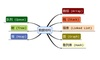数据结构 订阅